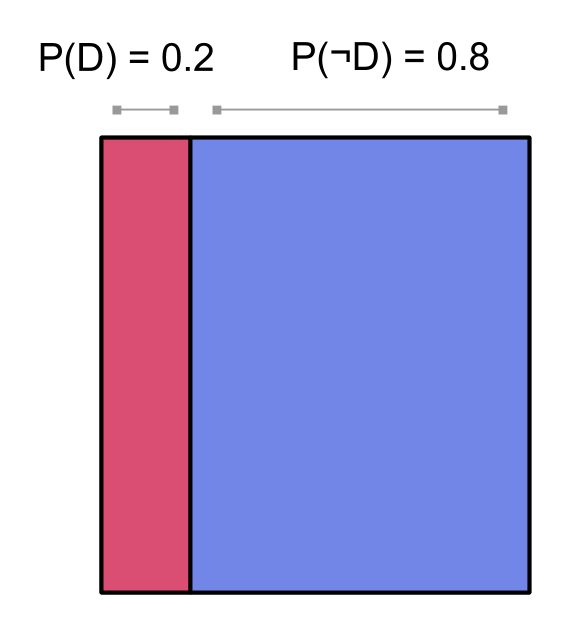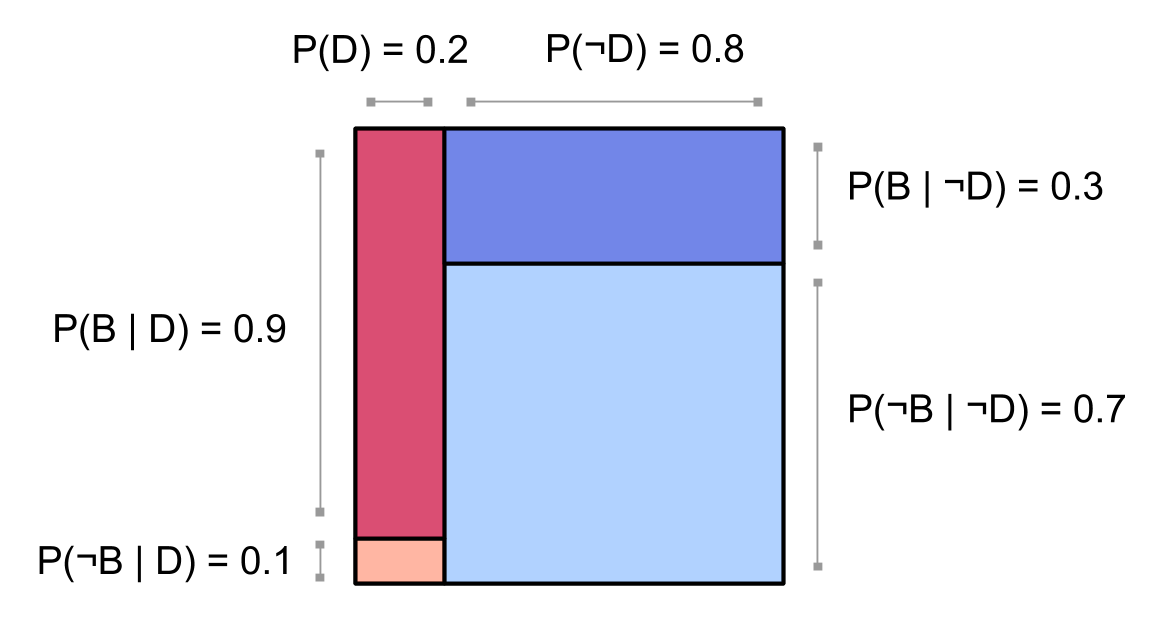# Square visualization of probabilities on two events: (example) Diseasitis

$$\newcommand{\bP}{\mathbb{P}}$$

From the Diseasitis:

You are screening a set of patients for a disease, which we’ll call Diseasitis. Based on prior epidemiology, you expect that around 20% of the patients in the screening population will in fact have Diseasitis. You are testing for the presence of the disease using a tongue depressor containing a chemical strip. Among patients with Diseasitis, 90% turn the tongue depressor black. However, 30% of the patients without Diseasitis will also turn the tongue depressor black. Among all the patients with black tongue depressors, how many have Diseasitis?

It seems like, since Diseasitis very strongly predicts that the patient has a black tongue depressor, it should be the case that the conditional probability $$\bP( \text{Diseasitis} \mid \text{black tongue depressor})$$ is big. But actually, it turns out that a patient with a black tongue depressor is more likely than not to be completely Diseasitis-free.

Can we see this fact at a glance? Below, we’ll use the square visualization of probabilities on two events to draw pictures and use our visual intuition.

To introduce some notation: our prior probability $$\bP(D)$$ that the patient has Diseasitis is $$0.2$$. We think that if the patient is sick $$(D)$$, then it’s 90% likely that the tongue depressor will turn black $$(B)$$: we assign conditional probability $$\bP(B \mid D) = 0.9$$. We assign conditional probability $$\bP(B \mid \neg D) = 0.3$$ that the tongue depressor will be black even if the patient isn’t sick. We want to know $$\bP(D \mid B)$$, the posterior probability that the patient has Diseasitis given that we’ve seen a black tongue depressor.

If we wanted to, we could solve this problem precisely using Bayes’ rule:

\begin{align} \bP(D \mid B) &= \frac{\bP(B \mid D) \bP(D)}{\bP(B)}\\ &= \frac{0.9 \times 0.2}{ \bP(B, D) + \bP(B, \neg D)}\\ &= \frac{0.18}{ \bP(D)\bP(B \mid D) + \bP(\neg D)\bP(B \mid \neg D)}\\ &= \frac{0.18}{ 0.18 + 0.24}\\ &= \frac{0.18}{ 0.42} = \frac{3}{7} \approx 0.43\ . \end{align}

So even if we’ve seen a black tongue depressor, the patient is more likely to be healthy than not: $$\bP(D \mid B) < \bP(\neg D \mid B) \approx 0.57$$.

Now, this calculation might be enlightening if you are a real expert at Bayes’ rule. A better calculation would probably be the odds ratio form of Bayes’s rule.

But either way, maybe there’s still an intuition saying that, come on, if the tongue depressor is such a strong indicator of Diseasitis that $$\bP(B \mid D) = 0.9$$, it must be that $$\bP(D \mid B) =big$$.

Let’s use the square visualization of probabilities to make it really visibly obvious that $$\bP(D \mid B) < \bP(\neg D \mid B)$$, and to figure out why $$\bP(B \mid D) = big$$ doesn’t imply $$\bP(D \mid B) =big$$.

We start with the probability of $$\bP(D)$$ (so we’re factoring our probabilities by $$D$$ first):Now let’s break up the red column, where $$D$$ is true and the patient has Diseasitis, into a block for the probability $$\bP(B \mid D)$$ that $$B$$ is also true, and a block for the probability $$\bP(\neg B \mid D)$$ that $$B$$ is false.

Among patients with Diseasitis, 90% turn the tongue depressor black.

That is, in 90% of the outcomes where $$D$$ happens, $$B$$ also happens. So $$0.9$$ of the red column will be dark ($B$), and $$0.1$$ will be light:However, 30% of the patients without Diseasitis will also turn the tongue depressor black.

So we break up the blue $$\neg D$$ column by $$\bP(B \mid \neg D) = 0.3$$ and $$\bP(\neg B \mid \neg D) = 0.7$$:Now we would like to know the probability $$\bP(D \mid B)$$ of Diseasitis once we’ve observed that the tongue depressor is black. Let’s break up our diagram by whether or not $$B$$ happens:Conditioning on $$B$$ is like only looking at the part of our distribution where $$B$$ happens. So the probability $$\bP(D \mid B)$$ of $$D$$ conditioned on $$B$$ is the proportion of that area where $$D$$ also happens:Here we can see why $$\bP(D \mid B)$$ isn’t all that big. It’s true that $$\bP(B,D)$$ is big relative to $$\bP(\neg B,D)$$, since we know that $$\bP(B \mid D)$$ is big (patients with Diseasitis almost always have black tongue depressors):But this ratio doesn’t really matter if we want to know $$\bP(D \mid B)$$, the probability that a patient with a black tongue depressor has Diseasitis. What matters is that we also assign a reasonably high probability $$\bP(B, \neg D)$$ to the patient having a black tongue depressor but nevertheless not suffering from Diseasitis:So even when we see a black tongue depressor, there’s still a pretty high chance the patient is healthy anyway, and our posterior probability $$\bP(D\mid B)$$ is not that high. Recall our square of probabilities:When asked about $$\bP(D\mid B)$$, we think of the really high probability $$\bP(B\mid D) = 0.9$$:Really, we should look at the part of our probability mass where $$B$$ happens, and see that a sizeable portion goes to places where $$\neg D$$ happens, and the patient is healthy:## Side note

The square visualization is very similar to frequency diagrams, except we can just think in terms of probability mass rather than specifically frequency. Also, see that page for waterfall diagrams, another way to visualize updating probabilities.